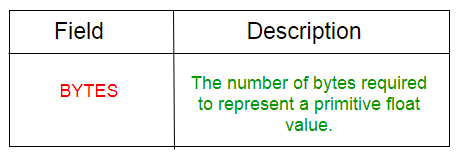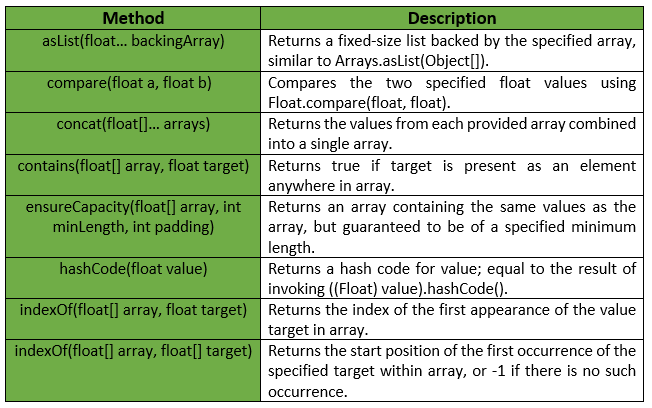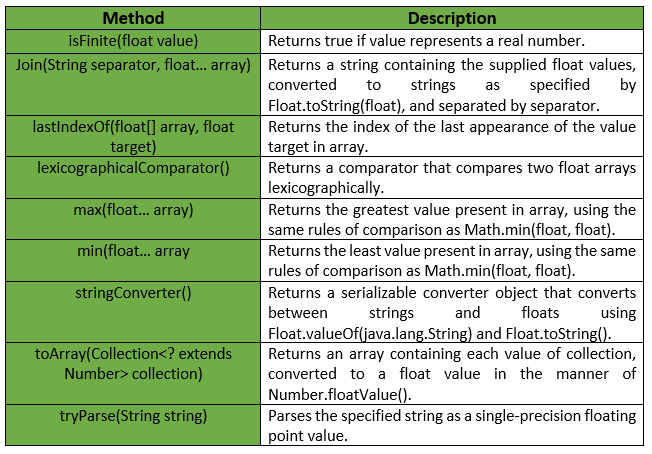# Floats Class | Guava | Java

Floats is a utility class for primitive type float. It provides Static utility methods pertaining to float primitives, that are not already found in either Float or Arrays.

Declaration :

```@GwtCompatible(emulated=true)
public final class Floats
extends Object
```

Below table shows the Field summary for Guava Floats Class :Some of the methods provided by Guava Floats Class are :Exceptions :

• min : IllegalArgumentException if array is empty.
• max : IllegalArgumentException if array is empty.
• ensureCapacity : IllegalArgumentException if minLength or padding is negative.
• toArray : NullPointerException if collection or any of its elements is null.

Below table shows some other methods provided by Guava Floats Class :Below given are some examples showing the implementation of methods of Guava Floats Class :
Example 1 :

 `// Java code to show implementation ` `// of Guava Floats.asList() method ` ` `  `import` `com.google.common.primitives.Floats; ` `import` `java.util.*; ` ` `  `class` `GFG { ` `    ``// Driver method ` `    ``public` `static` `void` `main(String[] args) ` `    ``{ ` `        ``float` `arr[] = { ``2``.6f, ``4``.6f, ``1``.2f, ``2``.4f, ``1``.5f }; ` ` `  `        ``// Using Floats.asList() method which ` `        ``// converts array of primitives to array of objects ` `        ``List myList = Floats.asList(arr); ` ` `  `        ``// Displaying the elements ` `        ``System.out.println(myList); ` `    ``} ` `} `

Output :

```[2.6, 4.6, 1.2, 2.4, 1.5]
```

Example 2 :

 `// Java code to show implementation ` `// of Guava Floats.toArray() method ` ` `  `import` `com.google.common.primitives.Floats; ` `import` `java.util.*; ` ` `  `class` `GFG { ` `    ``// Driver method ` `    ``public` `static` `void` `main(String[] args) ` `    ``{ ` `        ``List myList = Arrays.asList(``2``.6f, ``4``.6f, ``1``.2f, ``2``.4f, ``1``.5f); ` ` `  `        ``// Using Floats.toArray() method which ` `        ``// converts a List of Floats to an ` `        ``// array of float ` `        ``float``[] arr = Floats.toArray(myList); ` ` `  `        ``// Displaying the elements ` `        ``System.out.println(Arrays.toString(arr)); ` `    ``} ` `} `

Output :

```[2.6, 4.6, 1.2, 2.4, 1.5]
```

Example 3 :

 `// Java code to show implementation ` `// of Guava Floats.concat() method ` ` `  `import` `com.google.common.primitives.Floats; ` `import` `java.util.*; ` ` `  `class` `GFG { ` `    ``// Driver method ` `    ``public` `static` `void` `main(String[] args) ` `    ``{ ` `        ``float``[] arr1 = { ``2``.6f, ``4``.6f, ``1``.2f }; ` `        ``float``[] arr2 = { ``2``.4f, ``1``.5f }; ` ` `  `        ``// Using Floats.concat() method which ` `        ``// combines arrays from specified ` `        ``// arrays into a single array ` `        ``float``[] arr = Floats.concat(arr1, arr2); ` ` `  `        ``// Displaying the elements ` `        ``System.out.println(Arrays.toString(arr)); ` `    ``} ` `} `

Output :

```[2.6, 4.6, 1.2, 2.4, 1.5]
```

Example 4 :

 `// Java code to show implementation ` `// of Guava Floats.contains() method ` ` `  `import` `com.google.common.primitives.Floats; ` ` `  `class` `GFG { ` `    ``// Driver method ` `    ``public` `static` `void` `main(String[] args) ` `    ``{ ` `        ``float``[] arr = { ``2``.6f, ``4``.6f, ``1``.2f, ``2``.4f, ``1``.5f }; ` ` `  `        ``// Using Floats.contains() method which ` `        ``// checks if element is present in array ` `        ``// or not ` `        ``System.out.println(Floats.contains(arr, ``2``.5f)); ` `        ``System.out.println(Floats.contains(arr, ``1``.5f)); ` `    ``} ` `} `

output :

```false
true
```

Example 5 :

 `// Java code to show implementation ` `// of Guava Floats.min() method ` ` `  `import` `com.google.common.primitives.Floats; ` ` `  `class` `GFG { ` `    ``// Driver method ` `    ``public` `static` `void` `main(String[] args) ` `    ``{ ` `        ``float``[] arr = { ``2``.6f, ``4``.6f, ``1``.2f, ``2``.4f, ``1``.5f }; ` ` `  `        ``// Using Floats.min() method ` `        ``System.out.println(Floats.min(arr)); ` `    ``} ` `} `

Output :

```1.2
```

Example 6 :

 `// Java code to show implementation ` `// of Guava Floats.max() method ` ` `  `import` `com.google.common.primitives.Floats; ` ` `  `class` `GFG { ` `    ``// Driver method ` `    ``public` `static` `void` `main(String[] args) ` `    ``{ ` `        ``float``[] arr = { ``2``.6f, ``4``.6f, ``1``.2f, ``2``.4f, ``1``.5f }; ` ` `  `        ``// Using Floats.max() method ` `        ``System.out.println(Floats.max(arr)); ` `    ``} ` `} `

Output :

```4.6
```

Whether you're preparing for your first job interview or aiming to upskill in this ever-evolving tech landscape, GeeksforGeeks Courses are your key to success. We provide top-quality content at affordable prices, all geared towards accelerating your growth in a time-bound manner. Join the millions we've already empowered, and we're here to do the same for you. Don't miss out - check it out now!